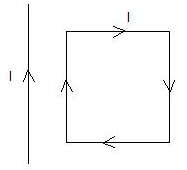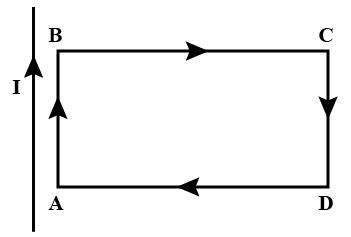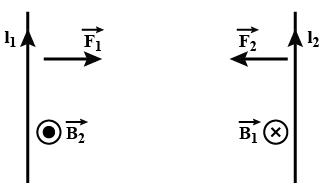Courses

# Test: Force On Current Carrying Wire

## 10 Questions MCQ Test Physics Class 12 | Test: Force On Current Carrying Wire

Description
This mock test of Test: Force On Current Carrying Wire for JEE helps you for every JEE entrance exam. This contains 10 Multiple Choice Questions for JEE Test: Force On Current Carrying Wire (mcq) to study with solutions a complete question bank. The solved questions answers in this Test: Force On Current Carrying Wire quiz give you a good mix of easy questions and tough questions. JEE students definitely take this Test: Force On Current Carrying Wire exercise for a better result in the exam. You can find other Test: Force On Current Carrying Wire extra questions, long questions & short questions for JEE on EduRev as well by searching above.
QUESTION: 1

### An electron and proton enter a magnetic field with equal velocities. Which one of them experiences a greater force?

Solution:

As charges and velocities are same
F=q(V×B)
So having the same magnitude of charge and same velocity, they'll experience the same magnitude of force.

QUESTION: 2

### Two thin long parallel wires separated by a distance b are carrying a current i ampere each. The magnitude of the force per unit length exerted by one wire on the other is

Solution:

Given, i1​=i2​=i
∴F=μ0​i2l​/2πb
Hence, force per unit length is F=μ0​i2​/2πb

QUESTION: 3

### The constant μo is called​

Solution:

The permeability of free space, μ0, is numerically equal to 4π x 10-7. For air and other non-magnetic materials, the absolute permeability is the same constant. For magnetic materials, absolute permeability is not a fixed constant but varies non-linearly with the flux density.

QUESTION: 4

A rectangular loop carrying a current I is situated near a long straight wire such that the wire is parallel to the one of the sides of the loop and is in a plane of the loop. If a steady current I is established in wire as shown in figure, the loop willSolution:

The long straight wire and side AB  carry current in the same direction, hence will attract each other.
The long straight wire and side CD carry current in the opposite direction, hence will repel each other.
Force on side BC  will be equal and opposite to force on side DA.
Since CD  is farther from the wire than AB,  the force of attraction on  AB  will exceed the force of repulsion on CD.
Hence, there will be a net force of attraction on the loop ABCD and it will move towards the wire.QUESTION: 5

In two current carrying conductors parallel currents________, anti parallel currents_________ .​

Solution:

parallel currents attract from the below figure.
and similarly, in antiparallel currents repel.parallel currents attract from the below figure.
and similarly, in antiparallel currents repel.QUESTION: 6

No force is exerted by magnetic field on a stationary

Solution:

Electric dipole at rest can be considered as charge particles at rest. hence, no magnetic field is applied on it.

QUESTION: 7

Along an infinitely long conductor carrying a current of 8 A we keep another conductor of length 5 m carrying a current of 3 A. Both the conductors are 10 cm apart. Find the force on small conductor.​

Solution:

I1=3A
I2=8A
l=5m
r=0.1m
F=?
Force per unit length on the small conductor is given by:
f= μo2I1I2/4πr
Total force on the length of small conductor is
F=fl
F= μo2I1I2 l/4πr
F=4πx10-7x2x3x8x5/4π x 0.1
F=2.4x10-4N

QUESTION: 8

We use _________ to find the direction of force when two current carrying conductors are kept parallel to each other.

Solution:

The direction of force (motion) of a current carrying conductor in a magnetic field is given by Fleming’s left hand rule.
It states that ‘ If we hold the thumb, fore finger and middle finger of the left hand perpendicular to each other such that the fore finger points in the direction of magnetic field, the middle finger points in the direction of current, then the thumb shows the direction of force (motion) of the conductor.

QUESTION: 9

The connecting wires of a battery of an automobile carry 200 A of current. Calculate the force per unit length between the wires if they are 50 cm long and 2 cm apart?​

Solution:

Current in both wires, I = 200 A
Distance between the wires, r = 2 cm = 0.02 m
Length of the two wires, l = 50 cm = 0.5 m
Force between the two wires is given by the relation,
F=μoI2/2πr
where, μo = permeability of free space = 4π x 10-7 TmA-1
So, F = [4π x 10-7 x (2002)]/[2π x 0.02]
⇒   F   =  0.4 Nm-1

QUESTION: 10

A 2 cm long copper wire having mass of 6 gm, dips in to two mercury pools to form a complete circuit. The wire is placed between the poles of a magnet which causes a field strength of 0.3 T. Find the initial upward acceleration of copper wire after 100 A of current is passed through the wire (g = 10 m/s2)

Solution:

ma=Bil−mg
a=0.3×100×2×10−2−10×6×10−3​/ m
a= 0.6−0.06​/6×10−3
=100−10
=90ms−2